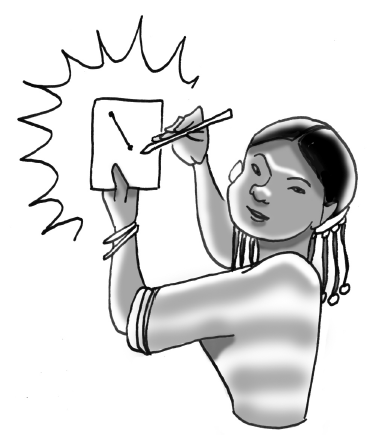Home > GB8I > Chapter cc43 > Lesson cc43.1.2 > Problem3-20

3-20.

Jamila wants to play a game called “Guess My Line”. She gives you the following hints: 3-20 HW eTool (Desmos) Homework Help ✎
“Two points on my line are $\it (1, 1)$ and $\it (2, 4)$.”

1. What is the slope of her line? A graph of the line may help.

2. What is the $y$intercept of her line?

$y$-intercept $= (0,−2)$

3. What is the equation of her line?

Use the rate of growth and the $y$-intercept to write an equation for the line. $y = mx + b$

Use the eTool below to complete the graph of the line and help solve each part.
Click the link at right for the full version of the eTool: Int1 3-20 HW eTool.